## 14.1.1 Problem Formulation

Motion planning under differential constraints can be considered as a variant of classical two-point boundary value problems (BVPs) . In that setting, initial and goal states are given, and the task is to compute a path through a state space that connects initial and goal states while satisfying differential constraints. Motion planning involves the additional complication of avoiding obstacles in the state space. Techniques for solving BVPs are unfortunately not well-suited for motion planning because they are not designed for handling obstacle regions. For some methods, adaptation may be possible; however, the obstacle constraints usually cause these classical methods to become inefficient or incomplete. Throughout this chapter, the BVP will refer to motion planning with differential constraints and no obstacles. BVPs that involve more than two points also exist; however, they are not considered in this book.

It is assumed that the differential constraints are expressed in a state transition equation,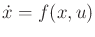, on a smooth manifold, called the state space, which may be a C-spaceor a phase space of a C-space. A solution path will not be directly expressed as in Part II but is instead derived from an action trajectory via integration of the state transition equation.

Let the action space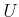be a bounded subset of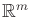. A planning algorithm computes an action trajectory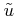, which is a function of the form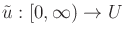. The action at a particular time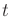is expressed as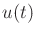. To be consistent with standard notation for functions, it seems that this should instead be denoted as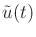. This abuse of notation was intentional, to make the connection to the discrete-stage case clearer and to distinguish an action,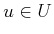, from an action trajectory. If the action space is state-dependent, thenmust additionally satisfy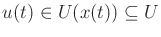. For state-dependent models, this will be assumed by default. It will also be assumed that a termination action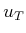is used, which makes it possible to specify all action trajectories over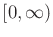with the understanding that at some time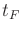, the termination action is applied.

The connection between the action and state trajectories needs to be formulated. Starting from some initial state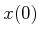at time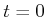, a state trajectory is derived from an action trajectoryas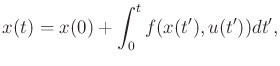(14.1)

which integrates the state transition equationfrom the initial condition. Let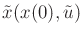denote the state trajectory over all time, obtained by integrating (14.1). Differentiation of (14.1) leads back to the state transition equation. Recall from Section 13.1.1 that ifis fixed, then the state transition equation defines a vector field. The state transition equation is an alternative expression of (8.14) from Section 8.3, which is the expression for an integral curve of a vector field. The state trajectory is the integral curve in the present context.

The problem of motion planning under differential constraints can be formulated as an extension of the Piano Mover's Problem in Formulation 4.1. The main differences in this extension are 1) the introduction of time, 2) the state or phase space, and 3) the state transition equation. The resulting formulation follows.

Formulation 14..1 (Motion Planning Under Differential Constraints)
1. A world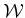, a robot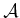(or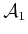,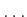,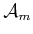for a linkage), an obstacle region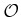, and a configuration space, which are defined the same as in Formulation 4.1.
2. An unbounded time interval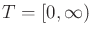.
3. A smooth manifold, called the state space, which may be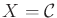or it may be a phase space derived fromif dynamics is considered; see Section 13.2. Let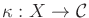denote a function that returns the configuration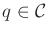associated with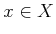. Hence,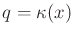.
4. An obstacle region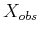is defined for the state space. If, then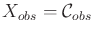. For general phase spaces,is described in detail in Section 14.1.3. The notation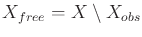indicates the states that avoid collision and satisfy any additional global constraints.
5. For each state, a bounded action space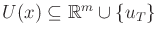, which includes a termination actionand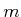is some fixed integer called the number of action variables. Letdenote the union of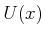over all.
6. A system is specified using a state transition equation, defined for everyand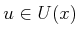. This could arise from any of the differential models of Chapter 13. If the termination action is applied, it is assumed that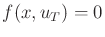(and no cost accumulates, if a cost functional is used).
7. A state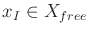is designated as the initial state.
8. A set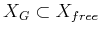is designated as the goal region.
9. A complete algorithm must compute an action trajectory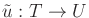, for which the state trajectory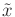, resulting from (14.1), satisfies: 1)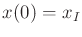, and 2) there exists some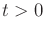for which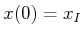and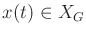.

Additional constraints may be placed on, such as continuity or smoothness over time. At the very least,must be chosen so that the integrand of (14.1) is integrable over time. Let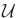denote the set of all permissible action trajectories over. By default,is assumed to include any integrable action trajectory. If desired, continuity and smoothness conditions can be enforced by introducing new phase variables. The method of placing integrators in front of action variables, which was covered in Section 13.2.4, can usually achieve the desired constraints. If optimizing a criterion is additionally important, then the cost functional given by (8.39) can be used. The existence of optimal solutions requires thatis a closed set, in addition to being bounded.

A final time does not need to be stated because of the termination action. As usual, onceis applied, cost does not accumulate any further and the state remains fixed. This might seem strange for problems that involve dynamics because momentum should keep the state in motion. Keep in mind that the termination action is a trick to make the formulation work correctly. In many cases, the goal corresponds to a subset ofin which the velocity components are zero. In this case, there is no momentum and hence no problem. If the goal region includes states that have nonzero velocity, then it is true that a physical system may keep moving afterhas been applied; however, the cost functional will not measure any additional cost. The task is considered to be completed afteris applied, and the simulation is essentially halted. If the mechanical system eventually collides due to momentum, then this is the problem of the user who specified a goal state that involves momentum.

The overwhelming majority of solution techniques are sampling-based. This is motivated primarily by the extreme difficultly of planning under differential constraints. The standard Piano Mover's Problem from Formulation 4.1 is a special case of Formulation 14.1 and is already PSPACE-hard . Optimal planning is also NP-hard, even for a point in a 3D polyhedral environment without differential constraints . The only known methods for exact planning under differential constraints in the presence of obstacles are for the double integrator system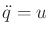, for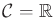and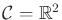.

Section 14.1.2 provides some perspective on motion planning problems under differential constraints that fall under Formulation 14.1, which assumes that the initial state is given and future states are predictable. Section 14.5 briefly addresses the broader problem of feedback motion planning under differential constraints.

Steven M LaValle 2020-08-14